# Metal sheet

How much m ^ 2 of sheet metal is needed to beat a box in the shape of a cube with an edge length of 50 cm, if we add 20% on the folds of the lid and walls?

x =  1.8 m2

### Step-by-step explanation: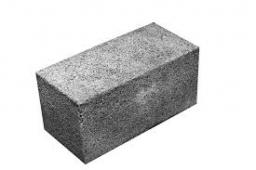Did you find an error or inaccuracy? Feel free to write us. Thank you!Tips to related online calculators
Do you want to convert area units?
Do you want to convert length units?

#### You need to know the following knowledge to solve this word math problem:

We encourage you to watch this tutorial video on this math problem:

## Related math problems and questions:

• Metal box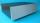How much metal we need for the production of box with dimensions 5 dm, 30 cm and a height of 1 m? Add 12% of the waste and folds.
• Wooden containerThe locksmith should cover the cube-shaped wooden container with a metal sheet inside. The outer edge of the container is 54cm. The wall thickness is 25 mm. The container has no lid. Calculate. How many sheets will be needed to cover it?
• Cuboid boxHow many m2 paper is needed for the sticking cuboid box of dimensions 50 cm, 40 cm and 30 cm? To the folds add one-tenth the area.
• Metal sheets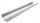How much metal sheet is needed to produce 8 gutters 4 m long and 12 cm in diameter? During production, joints calculate at 3% of total consumption.
• SeatHow much m² of fabric do we need to sew a 50cm-shaped cube-shaped seat if 10% of the material we add to the folds?
• GutterHow much metal is needed for production 60 pieces of gutter pipes with the diameter 17 cm and length of 6 m? The plate bends add 6% of the material.
• Gutter pipe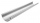How many m² of sheet metal is required to produce a 12 m long and 18 cm wide gutter, if 7% bend is required?
• Cylinder in cube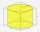Into a paper box in the shape of a cube with an edge of 10 cm is placed a can in the shape of a cylinder with a height of 10 cm and touching all the walls of the cube. What % of the volume of the cube can take up?
• Sheet of paperIs the sheet of paper measuring 18 and 25 cm enough for taping 9 cm cubes with an edge? (1 = yes, 0 = no)
• Roof coverAbove the pavilion with a square ground plan with a side length of a = 12 m is a pyramid-shaped roof with a height v = 4.5 m. Calculate how much m2 of sheet metal is needed to cover this roof if 5.5% of the sheet we must add for joints and waste.
• PrismRight-angled prism, whose base is a right triangle with leg a = 3 cm and hypotenuse c = 13 cm, has the same volume as a cube with an edge length of 3 dm. a) Find the height of the prism b) Calculate the surface of the prism c) What percentage of the cube'
• Cans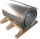How many m2 of metal sheet is needed to produce 20,000 cans in the shape of a cylinder with a base radius and a height of 5 cm.
• Surface of wallFind by what percentage the surface of the cube will decrease if we reduce the surface of each of its walls by 12%.
• The cube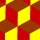The cube has an edge of 12 dm. The second cube has an edge exactly 20% longer. How many % is more water in the second cube than in the first cube, if the first cube is full to 3/4 and the second to 3/8?
• Cube wallThe surface of the first cube wall is 64 m2. The second cube area is 40% of the surface of the first cube. Determine the length of the edge of the second cube (x).
• Surface area 2Calculate how many % reduce the surface area of the cube is we reduced length of each edge by 10%.
• Pool tilesThe pool is 25m long, 10m wide, and 160cm deep. How many m2 of tiles will be needed on the walls and the pool? How many tiles are needed when 1 tile has a square shape with a 20cm side? How much does it cost when 1m2 of tiles costs 258 Kc?